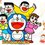# Prove that 2 + 2 = 4

Haha, I came across something quite funny on Math Stackexchange just now. :) Someone was asking for a proof that $2+2 =4$. Well, it's kind of intuitive because we see it in our every day life.

But let's go down deeper and explore this statement more rigourously. In other words, how do we really know for sure that that statement is true?!

Let me post that question to you now. Try it before you look at the link below. :)

Enjoy!Note by Happy Melodies
6 years, 5 months ago

This discussion board is a place to discuss our Daily Challenges and the math and science related to those challenges. Explanations are more than just a solution — they should explain the steps and thinking strategies that you used to obtain the solution. Comments should further the discussion of math and science.

When posting on Brilliant:

• Use the emojis to react to an explanation, whether you're congratulating a job well done , or just really confused .
• Ask specific questions about the challenge or the steps in somebody's explanation. Well-posed questions can add a lot to the discussion, but posting "I don't understand!" doesn't help anyone.
• Try to contribute something new to the discussion, whether it is an extension, generalization or other idea related to the challenge.

MarkdownAppears as
*italics* or _italics_ italics
**bold** or __bold__ bold
- bulleted- list
• bulleted
• list
1. numbered2. list
1. numbered
2. list
Note: you must add a full line of space before and after lists for them to show up correctly
paragraph 1paragraph 2

paragraph 1

paragraph 2

[example link](https://brilliant.org)example link
> This is a quote
This is a quote
    # I indented these lines
# 4 spaces, and now they show
# up as a code block.

print "hello world"
# I indented these lines
# 4 spaces, and now they show
# up as a code block.

print "hello world"
MathAppears as
Remember to wrap math in $$ ... $$ or $ ... $ to ensure proper formatting.
2 \times 3 $2 \times 3$
2^{34} $2^{34}$
a_{i-1} $a_{i-1}$
\frac{2}{3} $\frac{2}{3}$
\sqrt{2} $\sqrt{2}$
\sum_{i=1}^3 $\sum_{i=1}^3$
\sin \theta $\sin \theta$
\boxed{123} $\boxed{123}$

Sort by:

It's shocking that $2+2=4$ can be proven in only 2 pages, when it took 300 pages in Principia Mathematica by Russell and Whitehead to prove that $1+1=2$.

- 6 years, 5 months ago

300 pages!! I wonder what they wrote so much to prove that 1+1=2

- 6 years, 5 months ago

@Michael Mendrin I don't understand why it would need $300$ pages to proof that $1 + 1 =2$. By definition of multiplication $1 + 1 = 1 \times 2$ and since $1$ is the identity element for multiplication over all numbers (excluding zero) $1 + 1 = 1 \times 2 = 2 \times 1 = 2$. Can you explain to me why my proof is less "Rigorous"? Notice that I only used the definition of multiplication and identity element since both of them was used in "Rigorous" proof of $2 + 2 = 4$.

- 6 years, 5 months ago

Well the whole point of the proof is how do you prove the definition of multiplication, not just we know by the definition of multiplication that this multiplied by this is this.I think that is why you would need a 300 page proof right? @Michael Mendrin and @Siam Habib

- 6 years, 5 months ago

Yes, right. Russell and Whitehead were trying to put all of mathematics on a "rigorous logical foundation" with their Herculean Principia Mathematica effort, but the problem is much like what happens when you answer a child's question of "why?", he immediately asks the next "why?" in an infinite regression. Kurt Godel, after reading the efforts of Russell and Whitehead, finally came out with his [first of his incompleteness theorems] On Formally Undecidable Propositions in Principia Mathematica and Related Systems. He essentially showed that perhaps Russell and Whitehead were fruitlessly chasing their own tails, and that, after all, the idea of putting all of mathematics on a "consistent and complete" foundation was doomed from the beginning. Hence, we pretty much go back to simply saying, "1+1=2 because it just is.". @Siam Habib and @Mardokay Mosazghi

- 6 years, 5 months ago

Yea I think so.... Like referring to the proof of $-1 \times -1 = 1$ as I have posted as a comment above, the lemma proved that $0 = 0 \times 0$ which most of us think is common sense....

- 6 years, 5 months ago

I still wonder that how could they define all the notations for defining multiplication. =="

If the whole point of it is about defining multiplication, why did they want to write about proving $1+1=2$ instead?

- 6 years, 5 months ago

Wow! 300 pages??? Another link here to prove using only field axioms (using 0s) to prove that $-1 \times -1 = 1$. :D INTERESTING! :) (Refer to the first answer provided).

- 6 years, 5 months ago

Yes. Such is mathematics. Arithmetic requires so much information to compute.

- 6 years, 5 months ago

OMG 300 pages

- 6 years, 5 months ago

hey michael is this book available on internet ? i am eager to see this

- 6 years, 5 months ago

Such an easy question but no one can prove it!!!

- 6 years, 5 months ago

2+2=(2)+(1+1)=3+1=4 (This is a fact that adding 1 is moving right to the number line which increases the count by 1)

- 5 years, 7 months ago

Mind = Blown! :D

- 6 years, 5 months ago

Haha Ikr :)

- 6 years, 5 months ago